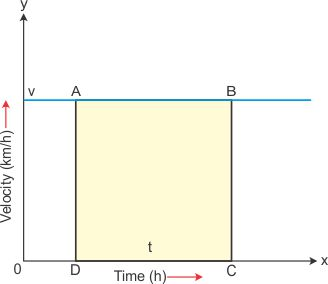1800-212-7858 (Toll Free)
9:00am - 8:00pm IST all days
8104911739

or

Thanks, You will receive a call shortly.
Customer Support

You are very important to us

022-62211530

Mon to Sat - 11 AM to 8 PM

Define uniformly accelerated motion. Also show that the area under a velocity-time graph gives the magnitude of displacement.

Asked by Topperlearning User 16th June 2016, 10:20 AM

If an object undergoes equal changes in velocity in equal intervals of time, then its motion is called uniformly accelerated motion.

A velocity-time graph gives the magnitude of displacement by calculating the area under the graph.

Consider the velocity-time graph of a body moving with uniform velocity.Area under the graph = area ABCD

= BC x AB

Assuming that line AB coincides at v m/s at the velocity axis and the DC = t s

Area under the graph = v x t

By definition: speed x time = magnitude of displacement.

Thus, the area under the velocity-time graph gives the magnitude of displacement.

Answered by Expert 16th June 2016, 12:20 PM
• 1
• 2
• 3
• 4
• 5
• 6
• 7
• 8
• 9
• 10

You have rated this answer 9/10